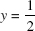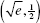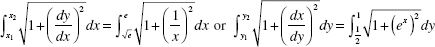# AP Calculus BC Question 268: Answer and Explanation

### Test Information

Question: 268

6. Find the length of the curve y = ln x between the points whereand y = 1.

• A. 0.53
• B. 0.86
• C. 1.18
• D. 1.36

(C) The endpoints of the arc areand (e,1). The arc length is given by